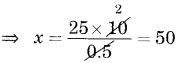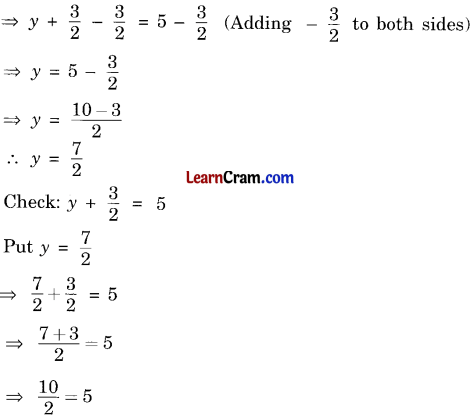# DAV Class 6 Maths Chapter 7 Brain Teasers Solutions

The DAV Class 6 Maths Solutions and DAV Class 6 Maths Chapter 7 Brain Teasers Solutions of Linear Equations offer comprehensive answers to textbook questions.

## DAV Class 6 Maths Ch 7 Brain Teasers Solutions

Question 1.
A. Tick (✓) the correct answer.
Twelve less than four times a number is 20, is represented by:
(i) 12 – 4x = 20
(ii) 20 = – 12 – 4x
(iii) 4x + 12 = 20
(iv) 4x – 12 = 20
4x – 12 = 20
Hence, (iv) is the correct option.

(b) Which of the following equations has x = 3 as a solution?
(i) x – 2 = 5
(ii) x + 2 = 5
(iii) 2x + 1 = 0
(iv) x + 4 = 6
On putting x = 3inx + 2 = 5,
we get 3 + 2 = 5, which is true.
Hence, (ii) is the correct option.

(c) The linear equation for 2 more than the sum of a and 4 is 8 will be:
(i) 2a + 4 = 8
(ii) 2(a + 4) = 8
(iii) (a + 4) + 2 = 8
(iv) a + 4 = 8
(iii) (a + 4) + 2 = 8
Hence, (iii) is the correct option.

(d) Which of the following is an equation of the given sentence?
Anjali is 5 years older than Nanjani.
(i) 5 + A = N
(ii) A + N = 5
(iii) N – 5 = A
(iv) A = N + 5
(iv) A = N + 5
Hence, (iv) is the correct option.

(e) Which of the following equations does not have a solution in integers?
(i) x + 1 = 1
(ii) x – 1 = 3
(iii) 2x + 1 = 6
(iv) 1 – x = 5
2x + 1 = 6
2x = 6 – 1
⇒ 2x = 5
x = $$\frac{5}{2}$$, which is not an integer.
Hence, (iii) is the correct option.(a) If x + 5 = 7, then 2x – 3 = ?
x + 5 = 7 (given)
∴ x = 7 – 5 = 2
Then, 2x – 3 = 2(2) – 3 = 4- 3 = 1

(b) What is the solution of the equation 5x + 5 = – 40?
5x + 5 = -40
5x = -40 – 5
5x = -45
x = $$\frac{-15}{5}$$ = -9

(c) If 0.5y = 1.5, find y.
0.5y = 1.5
y = $$\frac{1.5}{0.5}=\frac{15}{5}$$ = 3

(d) Check if p = 4 is the solution of $$\frac{1}{2}$$(p + 3) = 7.
On putting p = 4, we get
$$\frac{1}{2}$$(4 + 3) = $$\frac{1}{2}$$ × 7 = $$\frac{7}{2}$$
$$\frac{7}{2}$$ ≠ 7

(e) If $$\frac{x+2.4}{2}$$ = 3, then find x.
$$\frac{x+2.4}{2}$$ = 3
x + 2.4 = 6
x = 6 – 2.4
or x = 3.6

Question 2.
Write any five linear equations:
(i) x + 5 = 3
(ii) 2x + 3 = 7
(iii) 5x – 1 = 2x + 3
(iv) x + 1 = 2x – 3
(v) 2x + 5 = -4

Question 3.
Which side of the following equations is binomial?
(a) 2x + 1 = 7
(b) $$\frac{4}{5}$$y + 2 = 4
(c) 2(x + 3) = 8
(a) LHS (2x + 1) is binomial
(b) LHS ($$\frac{4}{5}$$y + 2) is binomial
(c) LHS 2(x + 3) is binomialQuestion 4.
Convert into linear equations:
(a) Thrice a number decreased by 5 is 9
(b) A number multiplied by two is 3 more than the number itself
(c) Twice a number subtracted from 13 is 5
(d) One-ninth of a number added to one is 11.
(a) 3x – 5 = 9
(b) 2x = x + 3
(c) 13 – 2x = 5
(d) $$\frac{x}{9}$$ + 1 = 11

Question 5.
Solve the following equations
(a) 4x – 3 = 2x + 1
4x – 3 = 2x + 1
⇒ 4x -3 + 3 = 2x + l + 3 (Adding 3 to both sides)
⇒ 4x = 2x + 4
⇒ 4x – 2x = 4 (Shifting 2x to LHS)
⇒ 2x = 4
⇒ $$\frac{2 x}{2}=\frac{4}{2}$$ (Dividing both sides by 2)
∴ x = 2

(b) 2(x – 5) = 10
⇒ 2(x – 5) = 10
⇒ $$\frac{2(x-5)}{2}=\frac{10}{2}$$ (Dividing both sides by 2)
⇒ x – 5 = 5
⇒ x – 5 + 5 = 5 + 5 (Adding 5 to both sides)
∴ x = 10

(c) $$\frac{x+8}{2}$$ + 8 = 4
⇒ $$\frac{x+8}{2}$$ × 2 = 4 × 2 (Multiplying both sides by 2)
⇒ x + 8 = 8
⇒ x + 8 – 8 = 8 – 8 (Adding -8 to both sides)
∴ x = 0

(d) 0.5x = 25
⇒ $$\frac{0.5 x}{0.5}=\frac{25}{0.5}$$ (Dividing both sides by 0.5)∴ x = 50

Question 5.
Solve the following equations and check the solutions:
(a) 1 – 4 x = -11
1 – 4x = -11
⇒ -1 + 1- 4x = -11- 1 (Adding —1 to both sides)
⇒ -4x = -12
⇒ $$\frac{-4 x}{-4}=\frac{-12}{-4}$$(Dividing both sides by -4)
∴ x = 3

Check: 1 – 4x = -11
Putting x = 3
1 – 4 × 3 = -11
⇒ 1 – 12 = -11
∴ -11 = -11 (Hence Verified)

(b) y + $$\frac{3}{2}$$ = 5∴ 5 = 5 (Hence Verified)

Question 6.
Complete the following statements with correct terms and signs:
(a) To solve the equation x + 3 = 8, we first add _____ to both sides.
-3

(b) To solve the equation 9y = 72, we _____ LHS and RHS by 9.
divide

(c) The solution of = 5 is _____
z = 15

(d) To solve the equation 2x – 3 = 6, we first add ______ to both sides and then divide both sides by _____.
3, 2

(e) The degree of variable in a linear equation is _____
1Question 1.
Solve the following equations and check.
(a) $$\frac{x-1}{2}$$ = 3 = 3
$$\frac{x-1}{2}$$ = 3 = 3
$$\frac{x-1}{2}$$ x 2 = 3 x 2 (Multiplying both sides by 2)
⇒ x – 1 = 6
⇒ x – 1 + 1 = 6 + 1 (Adding 1 to both sides)
x = 7
Check: $$\frac{x-1}{2}$$ = 3
Put x = 7
$$\frac{7-1}{2}$$ = 3
⇒ $$\frac{6}{2}$$ = 3
∴ 3 = 3 (Hence Verified)

(b) $$\frac{2 x+5}{3}$$ = 7
$$\frac{2 x+5}{3}$$ × 3 = 7 × 3
(Multiplying both sides by 3)
⇒ 2x + 5 = 21
⇒ 2x + 5 – 5 = 21- 5 (Adding -5 to both sides)
⇒ 2x = 16
⇒ $$\frac{2 x}{2}=\frac{16}{2}$$ (Dividing both sides by 2)
x = 8
Check: $$\frac{2 \times 8+5}{3}$$ = 7
Put x = 8
$$\frac{2 \times 8+5}{3}$$ = 7
$$\frac{16+5}{3}$$ = 7
$$\frac{21}{3}$$ = 7

Question 2.
Find the numerical coefficients in the following terms:
(a) –$$\frac{2}{3}$$xy
(b) –$$\frac{1}{2}$$x<sup>2</sup>y
(c) -5pq
(d) -p
(e) 0
(a) –$$\frac{2}{3}$$
(b) –$$\frac{1}{2}$$
(c) -5
(d) -1
(e) 0

Question 3.
Convert into linear equations:
(a) 5 is added to twice a number gives 17.
(b) Two-third of a number is added to itself gives 15.
(c) Twice a number subtracted from 13 is 5.
(d) One-eighth of a number is 2.
(a) 2x + 5 = 17
(b) $$\frac{2}{3}$$x + x = 15
(c) 13 – 2x = 5
(d) $$\frac{1}{8}$$x = 2

Question 4.
Convert the following equations in statements:
(a) 2x + 5 = 11
(b) x + 5 = 15
(c) $$\frac{x}{2}$$ – 5 = 7
(d) $$\frac{x}{2}$$ – $$\frac{x}{3}$$ = 1
(e) $$\frac{2y}{3}$$ = 4
(f) 7y = 21
(a) 5 is added to twice a number is 11.
(b) A number more than 5 is 15.
(c) 5 less than half of a number is 7.
(d) Difference between half and one-third of a number is 1.
(e) Two-third of a number is 4.
(f) Seven times a number is 21.

Question 5.
Fill in the blanks:
(a) To solve 3x + 5 = 10, we first add to both sides and then divide by _____
– 5, 3

(b) The solution of = 6 is _____
(d) The number which satisfy the equation 3x + $$\frac{1}{2}=\frac{7}{2}$$ is _____